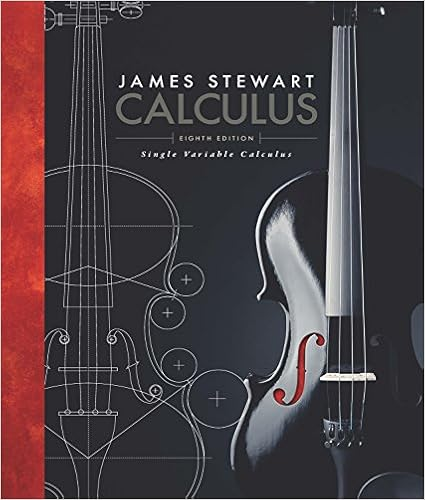# E using technology appropriately estimate the values

• Notes
• 22

This preview shows page 7 - 12 out of 22 pages.

##### We have textbook solutions for you!
The document you are viewing contains questions related to this textbook.The document you are viewing contains questions related to this textbook.
Chapter 4 / Exercise 46
Single Variable Calculus
StewartExpert Verified
(e) Using technology appropriately, estimate the values of F(5) and F(10)through appropriateRiemann sums.(f) Sketch an accurate graph of y=F(x)on the righthand axes provided, and clearly label thevertical axes with appropriate scale.
##### We have textbook solutions for you!
The document you are viewing contains questions related to this textbook.The document you are viewing contains questions related to this textbook.
Chapter 4 / Exercise 46
Single Variable Calculus
StewartExpert Verified
3. Evaluate each of the following derivatives and definite integrals. Clearly cite whether you usethe First or Second FTC in so doing.(a) ddxet4xdt(b) ddt2xt41+t4dt(c) ddxcost3()1dt(d) ddt3xln(1+t2)dt(e) ddxsint2()x3dt(Hint: Let F(x)=sint2()xdtand observe that this problem is asking you to evaluateddxF(x3).2x44
Section 5.3 – Integration by SubstitutionPreview Activity:Earlier, we learned the Chain Rule and how it can be applied to find the derivative of acomposite function. In particular, if uis a differentiable function of x, and fis x4+x2dxadifferentiable function of u(x), then ddxf(u(x))[]=f'(u(x))u'(x)In words, we say that the derivative of a composite function c(x)=f(u(x)), where fconsidered the “outer” function and i the “inner” function, is “the derivative of the outerfunction, evaluated at the inner function, times the derivative of the inner function.”(a) For each of the following functions, use the Chain Rule to find the function’s derivative. Besure to label each derivative by name (e.g., the derivative of g(x)should be labeled g'(x)i. g(x)=e3ii. h(x)=sin(5x+1)2x.is).x
)iv. q(x)=(27x)4
x(b) For each of the following functions, use your work in (a) to help you determine the generalantiderivative1 of the function. Label each antiderivative by name (e.g., the antiderivative of should be called M). In addition, check your work by computing the derivative of each proposedantiderivative.i. m(x)=e3ii. n(x)=cos(5x+1)iii. s(x)=11+42iv. v(x)=(27x)mxx3
x(c) Based on your experience in parts (a) and (b), conjecture an antiderivative for each of thefollowing functions. Test your conjectures by computing the derivative of each proposedantiderivative.i. a(x)=cosπx(ii. b(x)=4x+7()11iii. c(x)=xex)2
Activities:1. Evaluate each of the following indefinite integrals. Check each antiderivative that you find bydifferentiating:(a) sin(83x)dx(b) sec2(4x)dx(c) 111x9dx(d) csc(2x+1)cot(2x+1)dx(e) 116x2dx(f) 5xdx1
2. Evaluate each of the following indefinite integrals by using these steps:• Find two functions within the integrand that form (up to a possible missing constant) afunction-derivative pair;• Make a substitution and convert the integral to one involving uand du• Evaluate the new integral in u• Convert the resulting function of uback to a function of xby using your earlier substitution;• Check your work by differentiating the function of x. You should come up with the integrandoriginally given.;;
•••